# Linear Equations Quadratic Equations Proportion Simultaneous Equations Inequalities

• Slides: 27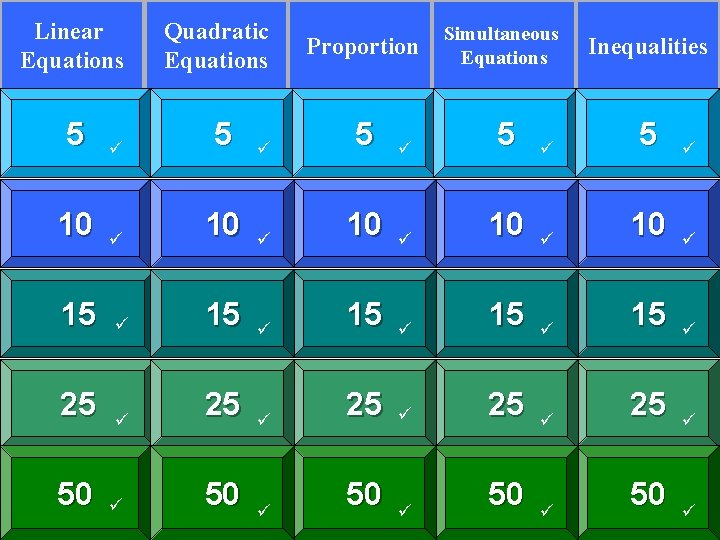Linear Equations Quadratic Equations Proportion Simultaneous Equations Inequalities 5 5 5 10 10 10 15 15 15 25 25 25 50 50 50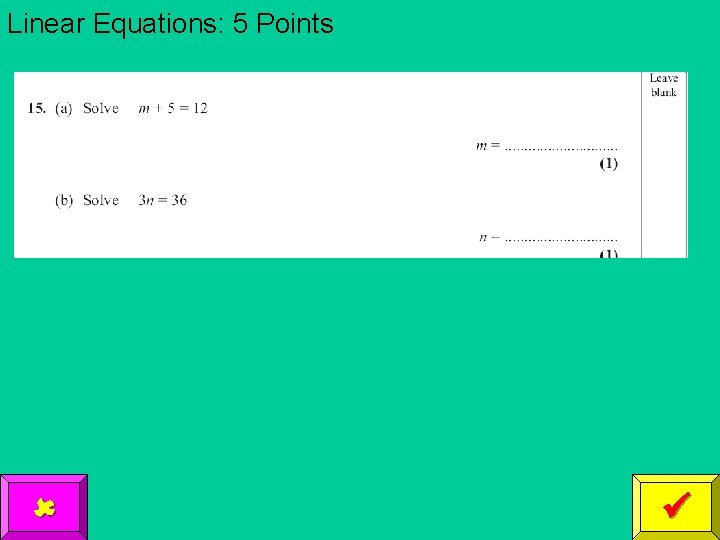Linear Equations: 5 PointsProportion: 5 Points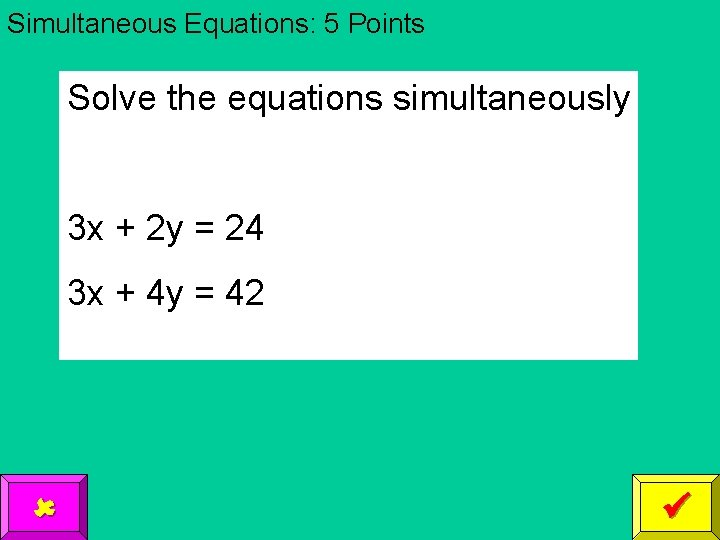Simultaneous Equations: 5 Points Solve the equations simultaneously 3 x + 2 y = 24 3 x + 4 y = 42Inequalities: 5 Points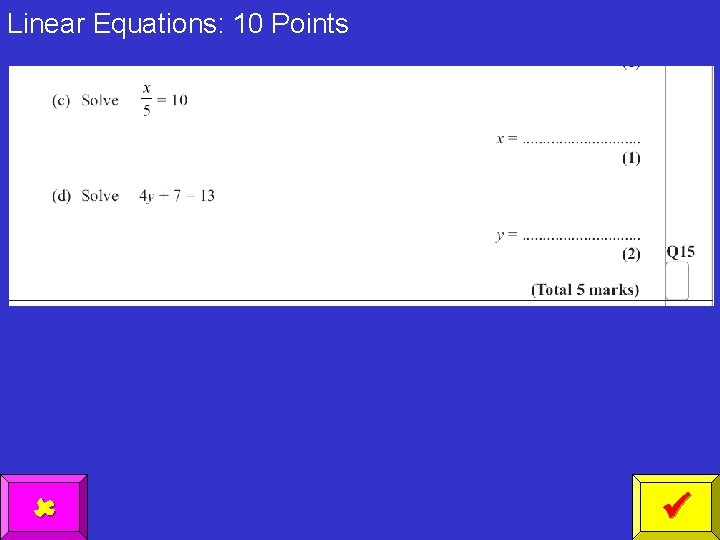Linear Equations: 10 PointsProportion: 10 Points y is proportional to x When y = 24, x = 6 What is the value of y when x = 2 (2 marks)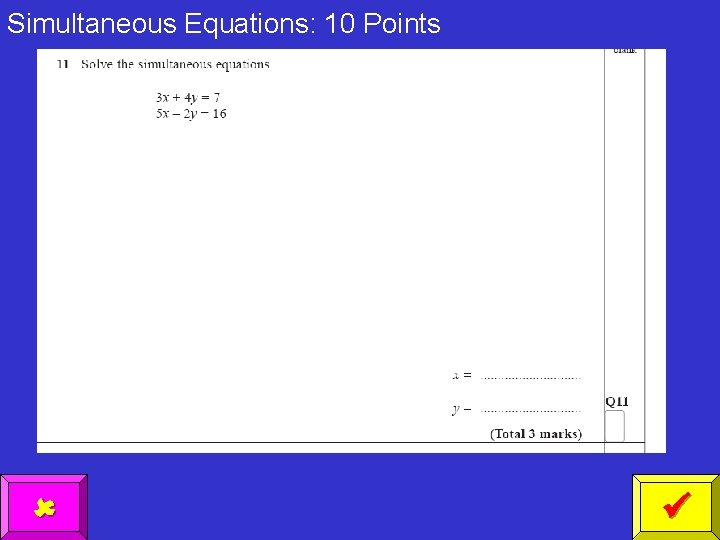Simultaneous Equations: 10 Points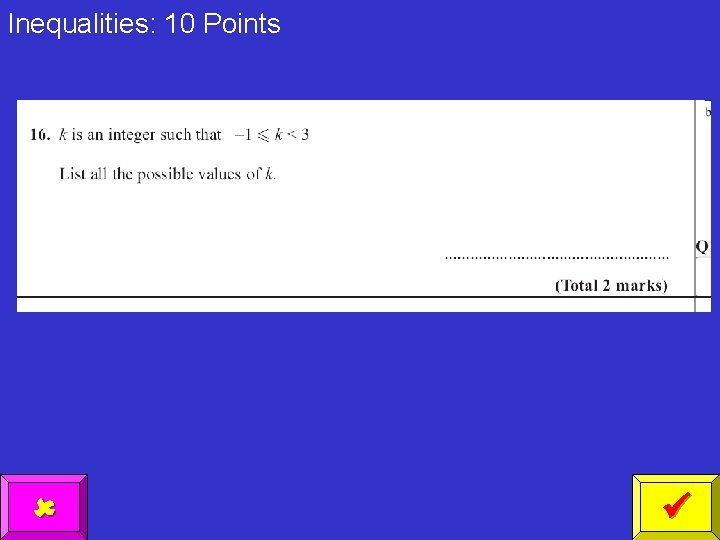Inequalities: 10 Points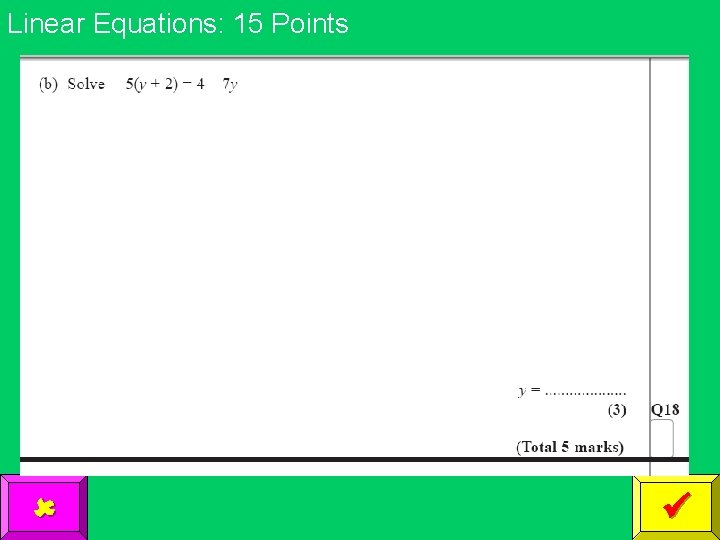Linear Equations: 15 Points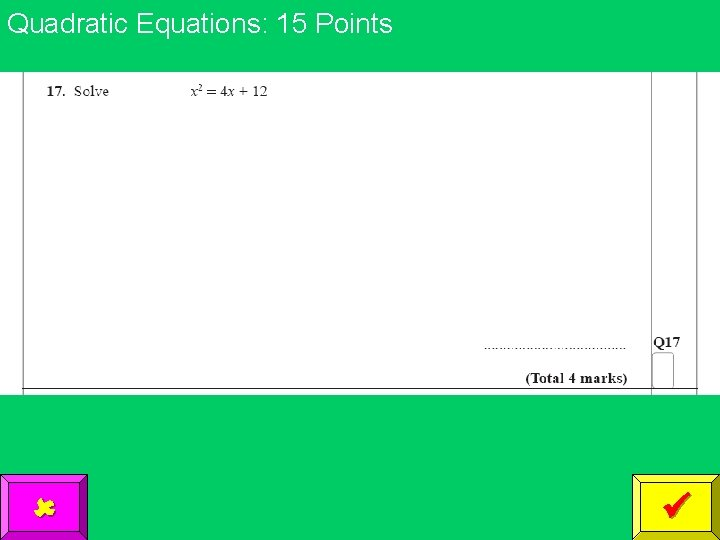Proportion: 15 Points y is proportional to x When y = 20, x = 5 What is the value of x when y = 36 (2 marks)Simultaneous Equations: 15 PointsInequalities: 15 PointsLinear Equations: 25 Points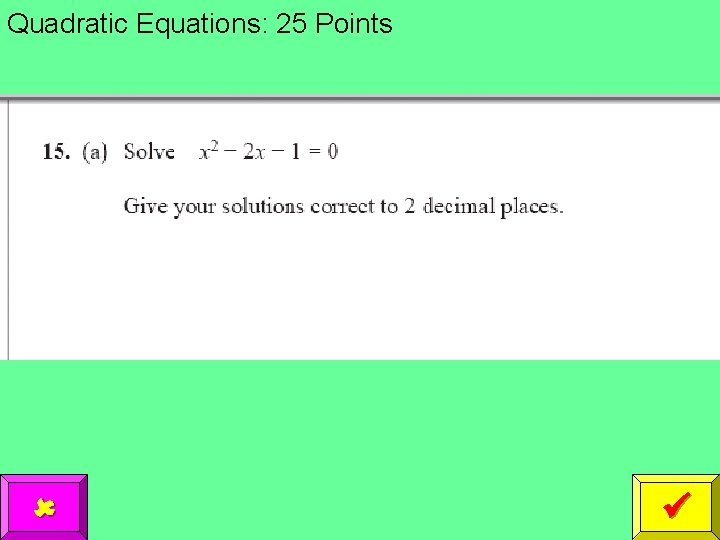Proportion: 25 PointsSimultaneous Equations: 25 Points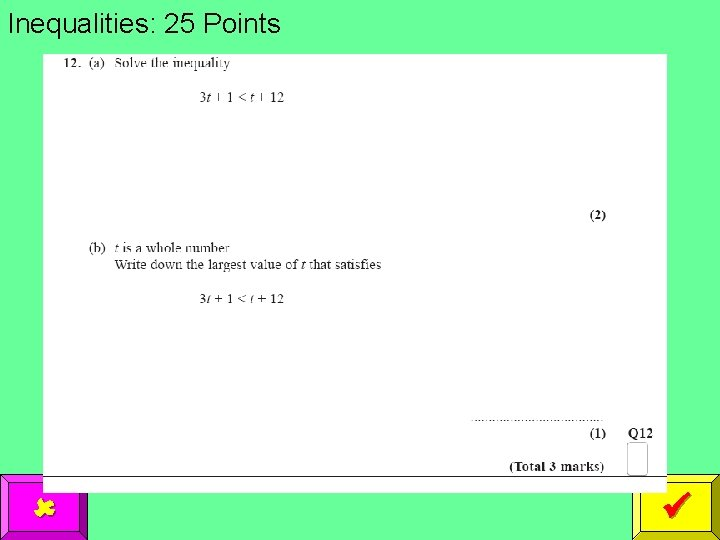Inequalities: 25 Points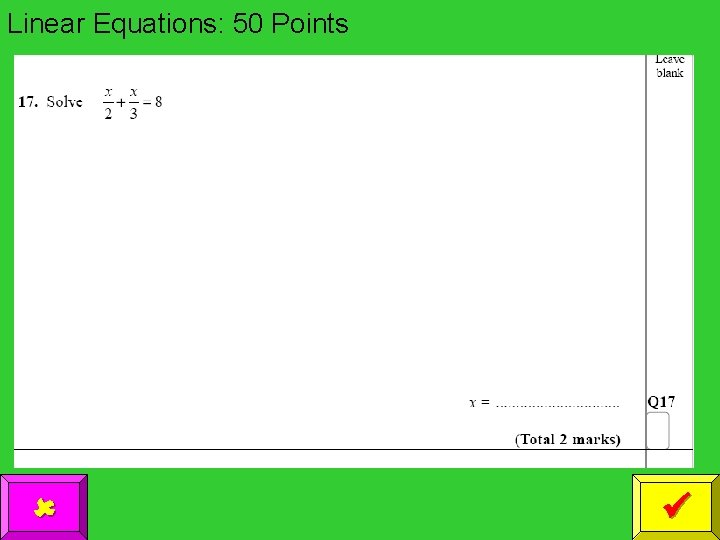Linear Equations: 50 PointsProportion: 50 Points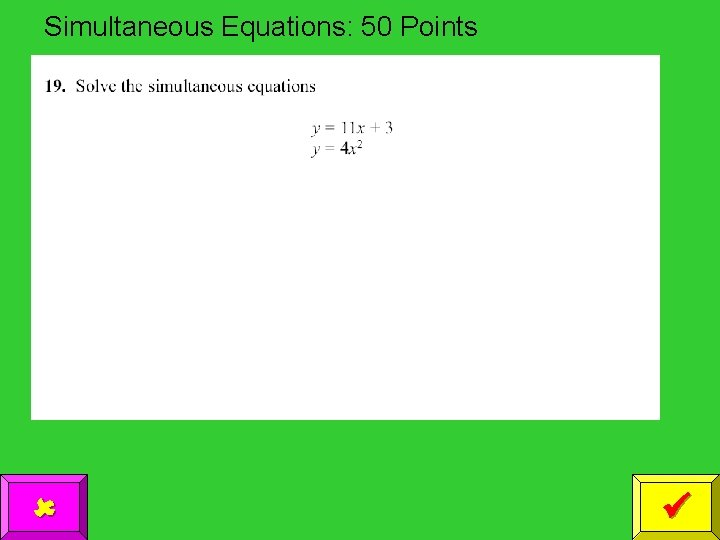Simultaneous Equations: 50 PointsInequalities: 50 Points Solve the following inequality x 2 + 3 x + 2 < 0